## Scripting

In a manner similar to using Matlab/Octave/R/Scilab, we may use SuanShu in an interactive or a scripting environment. This is most appropriate for research, data analysis and rapid prototyping. Often using a script language, the numerical problems may be expressed and solved in a reduced number of code lines as compared to using Java. Also, the users can get immediate feedback for each line execution, without having to first finish the whole program.

Here is a screenshot of using SuanShu in Groovy.# Tools

There are a number of ways to use SuanShu in a scripting environment. We recommend the following.

There are a number of advantages to using SuanShu over other mathematics scripting languages such as Matlab/Octave/R/Scilab. In the following comparisons, we are illustrating the pros and cons using R, but the arguments probably carry over to the other mathematics scripting languages.

## No Language Pollution

There is no need to learn and use a very specialized and proprietary language. In general, the proliferation (pollution) of languages causes confusion. There are very established programming languages that junior students and professional programmers already know. The most notable examples are Java, C# and C++. They are general enough to solve any computationally feasible problems. It makes no sense to invent a new language just for an application. The consequences of language pollution are:

1. the users need to learn a new language just to use a new application, hence a barrier of entry; e.g., the R language to use R;
2. the heterogeneity of languages introduces unnecessary challenges when combining them to build one integrated system; the more the worse;
3. debugging a variety of languages is difficult;
4. the hosting applications are almost never written in Matlab/Octave/R/Scilab; we would thus have to pass a large amount of data back and forth between the applications (e.g., in Java) and the data processing code (e.g., R); this is a severe performance issue;
5. the specialized language has no use outside its environment; e.g., no one uses the R language outside the R computing environment.

Most programmers already know Java (or can convert from C# and C++ very easily). As SuanShu is written entirely in Java, there is no need to learn a new language to use it.

## Better Code

The “real” programming languages (e.g., Java, Groovy, Scala) are better designed than the specialized mathematics languages. They are invented by scientists who really understand programming languages. The modern languages support (or shall I say, demand) object-oriented code, inheritance, interface, anonymous class, closure, functional programming, an advanced memory model and management, automatic garbage collection and etc. In contrast, the popular mathematics languages have few, if not none, of these features. For example, the R language is a by-and-large procedural imperative C-like language. (Its object-oriented feature is nothing more like a struct in C. It should not be confused with the real object-oriented support in e.g., Java.) It might be acceptable a few decades ago when computer science was at its dawn but certainly not after modern programming languages have come a long way.

In a nutshell, the users of modern programming languages can write better code, in terms of correctness, readability, easiness to debug (maintainability), modularity (object-oriented).

Let’s illustrate our opinion by numerically evaluating this integral:In Matlab/Octave/R/Scilab, we typically would write something like this.

n = 10000000
sum = 0
for (x in seq(0, 1, 1/n)) {
sum = sum + x * x # (*)
}
value = sum / n


This would be a piece of perfectly acceptable code many decades ago when C was the most popular programming language. Judging from the modern software engineering perspective, we make one criticism: the mathematics concepts involved are not modular; they cannot be separated out. All this snippet does is to describe one particular procedure to integrate one particular function. There is no reusable code. For example,

• if you want to integrate another function, you would need to copy and paste this snippet then modify (*);
• the integrand cannot be manipulated (as a first class citizen); it cannot be evaluated without making a copy outside the integration for-loop; it cannot be passed to another operation, e.g., differentiation, or another way of doing integration, without copying and pasting the same code.

Here is a modern object-oriented way of doing integration using SuanShu in Groovy. Note that the integration algorithm is now independent of the integrand. The integrator can take different integrands, and the integrand is no longer tied to the integrator. This is not to mention that the code is a lot shorter. One statement is to define a Riemann integrator; another statement is to define the integrand. The two objects are independent.

I = new Riemann();//create an integrator
v = I.integrate(
['evaluate' : {x -> return x * x}] as UnivariateRealFunction,//integrate the function y = x^2
0, 1);//limit: [0, 1]


## Software Engineering Process

Not only does the modern languages like Java allow the users to produce more elegant code, they also come with a suite of software engineering tools and paradigms to improve the coding experience as well as the quality of end products.

First, the computer science community has escaped from the procedural imperative programming mindset to object-oriented programming. We have designed many modern programming techniques, most notably design patterns for effective programming. However, it is not clear if the legacy mathematics languages support inheritance and interface to the extent that we can code patterns using them.

The modern languages come with tools to ensure code correctness. For example, for each mathematics concept, SuanShu has a corresponding class(es). The JUnit tool allows us to systematically and automatically do regression tests on all the source code regularly and when there are code changes. The same goes true for Groovy, Scala and etc. In contrast, suppose we have 100s of R scripts that depend on each other. When we make code changes in one of them, it is not clear how to systematically and automatically make sure that the changes are correct for this particular R file and that all other scripts will work after the changes. Specifically, suppose we make a fundamental change in the way the coefficients in an ARMA model are estimated, will all other functions that depend on this ARMA fitting still work? Theoretically maybe yes, but practically often no (unless you always write bug free code).

Below is a screenshot of the result of SuanShu unit testing.## OPTIM

This is a collection of the advanced optimization algorithms.

• linear programming (LP)
• second order conic programming (SOCP)
• semi-definite programming (SDP)
• mixed linear integer programming (MIP)
• genetic algorithms (GA)
• differential evolution optimization (DE)

## STATS

This is a collection of advanced statistics algorithms.

• cointegration
• covariance selection
• factor analysis
• hidden Markov model (Rabiner)
• hidden Markov model (mixture models: Beta, Binomial, Exponential, Gamma, Log-Normal, Normal, Poisson)
• hypothesis testing
• Kalman filter
• LASSO (Least Absolute Shrinkage and Selection Operator)
• linear regression
• principal component analysis
• stochastic process simulation
• time series analysis

## Hypothesis Testing

• Kolmogorov-Smirnov
• D’Agostino
• Jarque-Bera
• Lilliefors
• Shapiro-Wilk
• One-way ANOVA
• T
• Kruskal-Wallis
• Siegel-Tukey
• Van der Waerden
• Wilcoxon rank sum
• Wilcoxon signed rank
• Breusch-Pagan
• Glejser
• Harvey-Godfrey
• White
• Bartlett
• Brown-Forsythe
• F
• Levene

## Linear Regression

• OLS regression
• logistic regression
• GLM regression
• family: Binomial, Gamma, Gaussian, Inverse Gaussian, Poisson
• quasi-family: Binomial, Gamma, Gaussian, Inverse Gaussian, Poisson
• GLM model selection

## Time Series Analysis

• various moving average filters
• univariate/multivariate time series analysis
• ARIMA modeling, simulation, fitting, prediction
• GARCH modeling, simulation, fitting, prediction
• Auto-Covariance computation

# The NM Dev Tutorials

The NM Dev tutorials are practical guides for those who would like to create mathematics applications using the NM Dev library. They include lessons with many complete and working examples. Groups of related lessons are organized into “trails”.

For the most up-to-date information on using the NM Dev library, please consult the Javadoc. Please check out the NM Forum, the place to discuss the NM Dev library, mathematics and programming. You are also encouraged to post your questions there to get help beyond documents.

Before you start these lessons, please make sure you have set up the development environment properly. You can find the details in the Setup Guide.

# Hello NM Dev

This tutorial walks you through a simple first example on using the NM Dev library. You will need to have a NM Dev project to follow the examples here. For details on how to create or download a NM Dev project, please read the Setup Guide.

We will create some matrices and vectors and do some simple linear algebra. Java is an object-oriented language. So, other than the primitive types, like int and double, all variables are objects, namely, instances of some object definitions called classes. For example, to have a matrix instance, a matrix variable, or simply a matrix, we create an instance of the Matrix class.

Java matrix
Matrix A1 = new DenseMatrix(
new double[][]{ // create a matrix
{1, 2, 3},
{4, 5, 6},
{7, 8, 9}
});

We can print out the Java matrix to check.

System.out.println(A1);

In NetBeans the short cut for System.out.println is to type “sout” (without the “”) and then hit the “Tab” key.

To fix the imports, hit “Ctrl+Shift+I”. There are multiple choices. Choose com.numericalmethod.suanshu.matrix.doubles.Matrix.Hit “F6” to run.

Vola! You will see this output.

[,1] [,2] [,3]
[1,] 1.000000, 2.000000, 3.000000,
[2,] 4.000000, 5.000000, 6.000000,
[3,] 7.000000, 8.000000, 9.000000,

Congratulations! You have just successfully created your first matrix using the NM Dev library. We are now ready to do more.

Let’s check whether this matrix has a full rank. We do

int rank1 = MatrixMeasure.rank(A1); // compute A1's rank
System.out.println(rank1); // rank1 is 2 implies a deficient matrix

The MatrixMeasure.rank method computes the numerical rank. For more information, see Compute the numerical rank of a matrix.

Hit “F6” to run.

We see that the rank of A1 is 2 so A1 is a rank deficient matrix. We can make it a full rank matrix by changing one entry.

A1.set(3, 3, 10); // change A1[3, 3] to 10

Note that NM Dev counts from 1!

Let’s try again.

rank1 = MatrixMeasure.rank(A1); // compute modified A1's rank
System.out.println(String.format("rank for the modified A1 = %d", rank1));

OK. A1 is now a full rank matrix. We can now find its inverse.

Matrix A2 = new Inverse(A1); // compute A1 inverse
System.out.println(A2);

Hit “Ctrl+Shift+I” to fix the imports. Hit “F6” to run. Here is the output.

3x3

[,1] [,2] [,3]
[1,]  -0.666667, -1.333333, 1.000000,
[2,]  -0.666667, 3.666667, -2.000000,
[3,]  1.000000, -2.000000, 1.000000,

It looks good. To make sure, we multiply them together.

Matrix A3 = A1.multiply(A2); // A3 = A1 %*% A2
/** In numerical computing, we are usually satisfied to compare equality up to a precision.*/
final double precision = 1e-14; // A3 and the 3x3 identity matrix are equal up to 14 decimal points
if (AreMatrices.equal(A3, new DenseMatrix(3, 3).ONE(), precision)) { // compare A3 to the 3x3 identity matrix
System.out.println("A2 is indeed A1's inverse");

Hit “Ctrl+Shift+I” to fix the imports. Hit “F6” to run.

OK. It works.

Let’s try a vector. We do

Vector y = new DenseVector(new double[]{ // create a vector
20, 10, 4
});

Hit “Ctrl+Shift+I” to fix the imports. There are multiple choices. Choose “com.numericalmethod.suanshu.vector.doubles.Vector”.We solve a linear equation of this form.

Ax = y

We need to set up a linear equation solver.

LinearSystemSolver solver = new LinearSystemSolver(A1); // set up the linear equation solver
Vector x = solver.solve(y); // solve A1 %*% x = y
System.out.println(x);

Hit “Ctrl+Shift+I” to fix the imports. There are multiple choices. Choose com.numericalmethod.suanshu.matrix.doubles.linearsystem.LinearSystemSolver.

x is a vector

[-22.666667, 15.333333, 4.000000]

# Setup Guide

This tutorial is on how to set up the development environment for programming with the NM Dev library (the Java version). In order to use the NM Dev library, you need to know some Java programming. Sun (or Oracle) provides excellent online tutorials on learning Java. You can find a lot of easy-to-read-and-follow lessons in Sun’s Java Tutorials.

## IDE

You will need an Integrated Development Environment (IDE) to program NM Dev. Although you may choose any IDE you like, we recommend Netbeans. You can download it from here.To learn about how to use NetBeans, you will find a quick start tutorial here. The most important skill for you to learn is debugging. This is especially true for those who are converting from Matlab and R and are used to printing out values. NetBeans debugger allows you to easily inspect values, place conditional breakpoints, pause and resume your program and much more. A quick Google search gives these articles.

We cannot emphasize enough that it is imperative that you must master using a debugger to make your programming experience productive and enjoyable.

Hotkey is another tool that will increase your programming productivity. My favorite hotkey is Ctrl+Shift+F (or Alt+Shift+f if you are using windows). It makes my code look pretty and neat. A list of hotkeys is found here. You can customize them to your own taste by going to Netbeans -> Preferences -> Keymap (or Tools -> Options -> Keymap for Windows/Linux).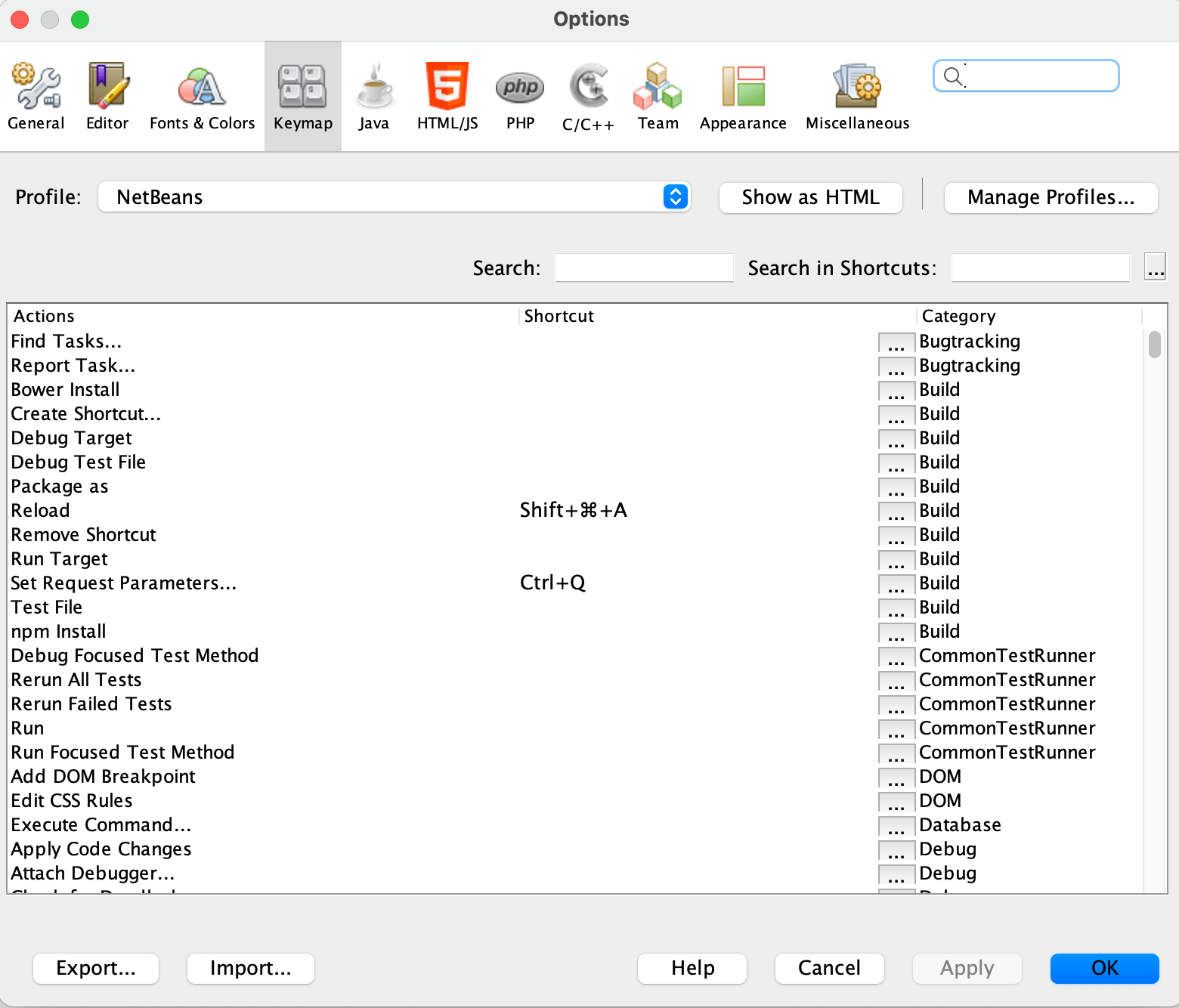## nmdev.jar

To program the NM Dev library, you will need a licensed copy of nmdev.jar (the actual file name depends on the version that you have). It is a compressed file that contains the NM Dev Java classes for numerical computing. To get a copy of the trial license, please email sales@nm.dev.nmdev-1.0.0.zip (13.1 MiB, 2,519 hits)

This section walks you through how to set up a NM Dev project. You may skip this section if you want to start programming NM Dev right away using the S2 IDE which comes in-built with NM Dev. Please read our Basic Trails to get started. If in the future you would like to update the NM Dev library for a newer version, you will need to come back to this section.

## Create a NM Dev project

To create a NM Dev project in NetBeans, open the NetBeans IDE, click File -> New Project… (Ctrl+Shift+N). In ‘categories’, choose ‘Java with Ant’; in projects, choose ‘Java Application’. Click ‘Next’.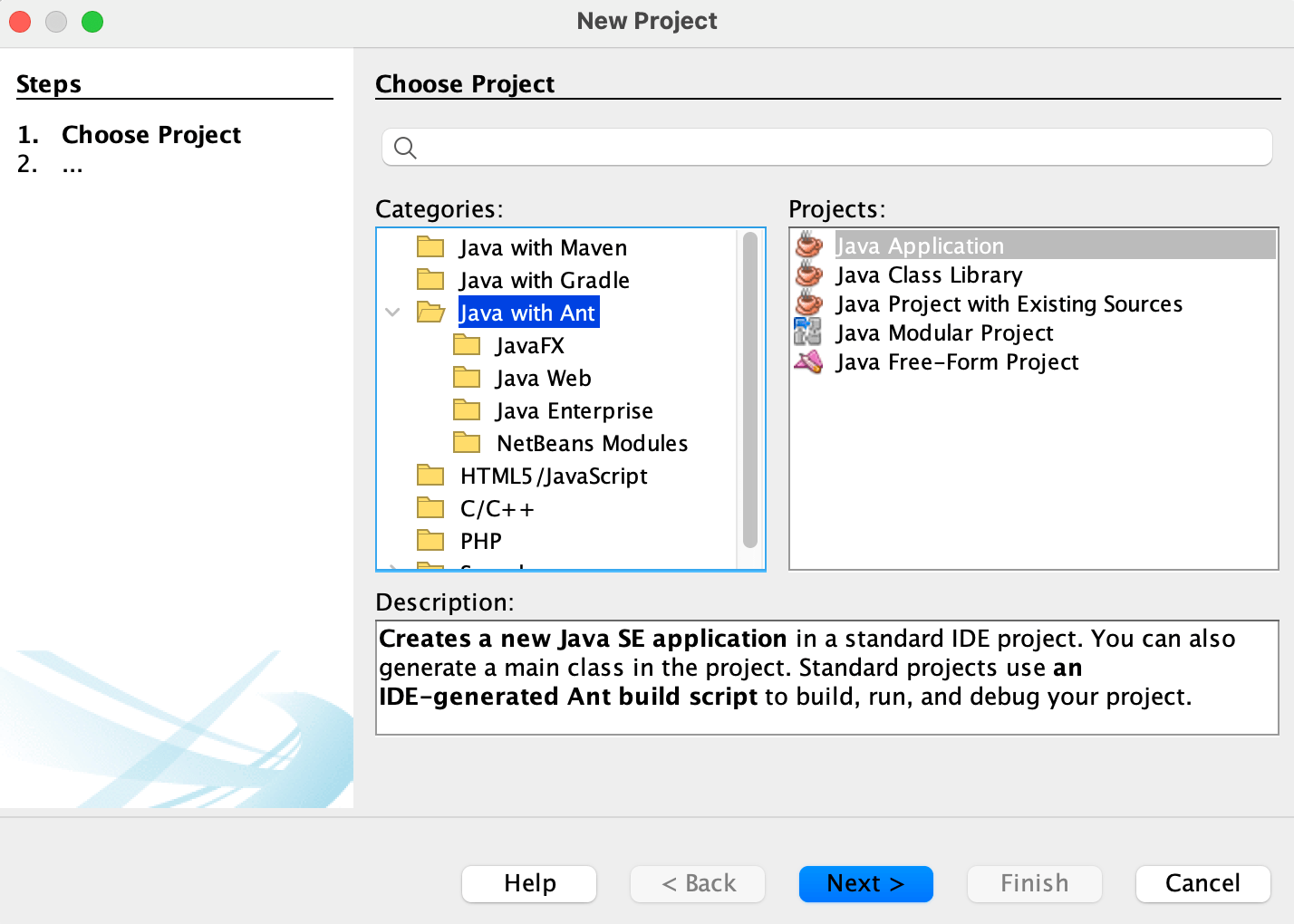In ‘Project Name’, type whatever you like, e.g., HelloNMDev. In ‘Project Location’, type where you would like to save your project. Make sure the box ‘Use dedicated Folder for Storing Libraries’ is checked.Then click ‘Finish’.We need to tell this Java project that we will be calling the NM Dev library. To do so, right click on the ‘Libraries’ Folder in the project tab and click ‘Add JAR/Folder’Browse to where you saved nmdev.jar. Select nmdev.jar and then hit ‘Open’.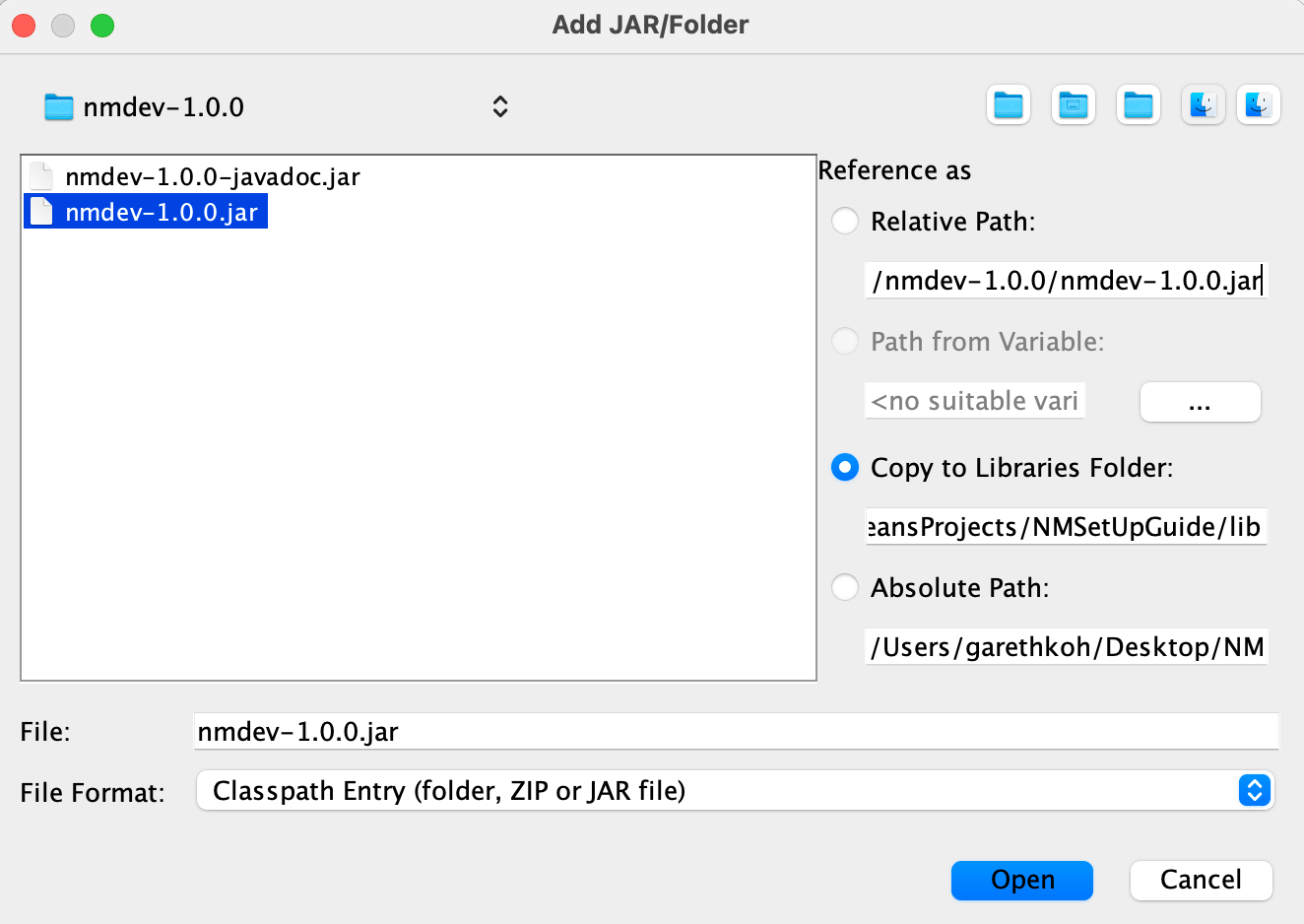To install the NM Dev javadoc in NetBeans, we need to associate the nmdev.jar with its javadoc. Right click the nmdev.jar file you just added and select ‘Edit’.In the popup window, under the ‘javadoc’ section, click ‘Browse’ and select the nmdev-javadoc.jar file. Then click ‘Open’.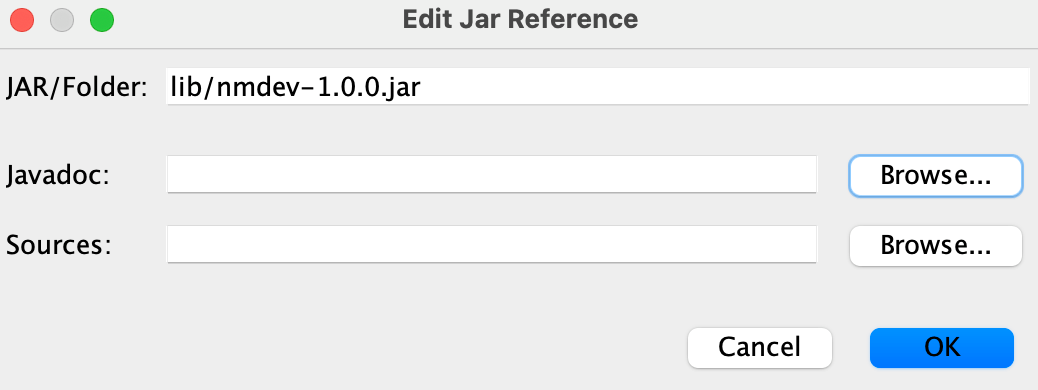Now, you have created an empty NM Dev project. Copy and paste these lines under “// TODO code application logic here“.

System.out.println(“Hello NM Dev”);

Matrix A1 = new DenseMatrix(new double[][]{//create a matrix

{1, 2, 1},

{4, 5, 2},

{7, 8, 1}

});

System.out.println(A1);

Matrix B = new Inverse(A1);//compute the inverse of A1

Matrix I = A1.multiply(B);//this should be the identity matrix

System.out.println(String.format(“%s * %s = %s (the identity matrix)”,

A1,

B,

I));

[/box]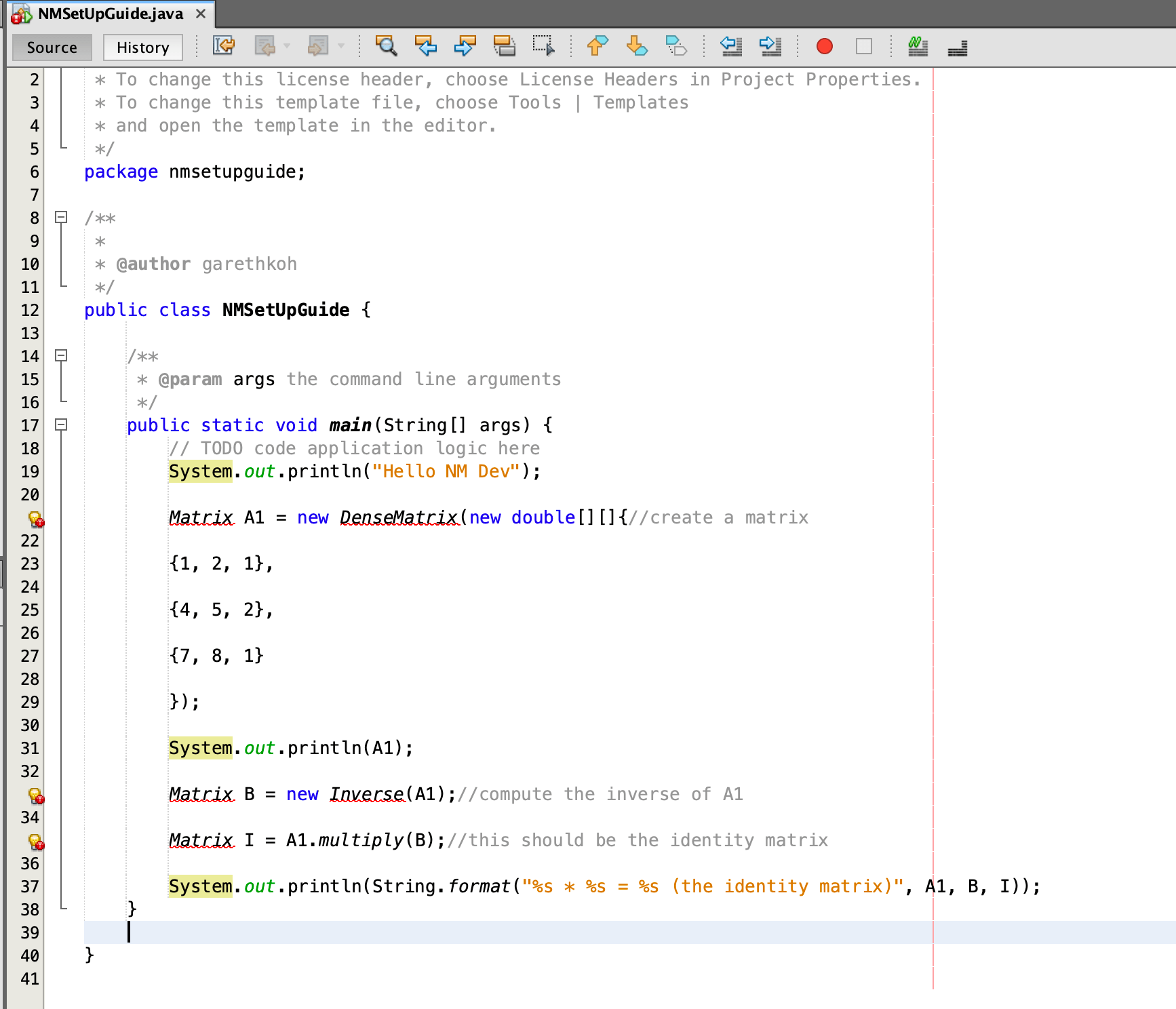Hit Command+Shift+I (or Ctrl+Shift+I on Windows/Linux) to fix the necessary imports. Make sure these statements appear at the top of your program. Else you can input them manually.

import dev.nm.algebra.linear.matrix.doubles.Matrix;
import dev.nm.algebra.linear.matrix.doubles.matrixtype.dense.DenseMatrix;
import dev.nm.algebra.linear.matrix.doubles.operation.Inverse;

[/box]

Hit Alt+Shift+F to beautify your code. Your code should look something like this.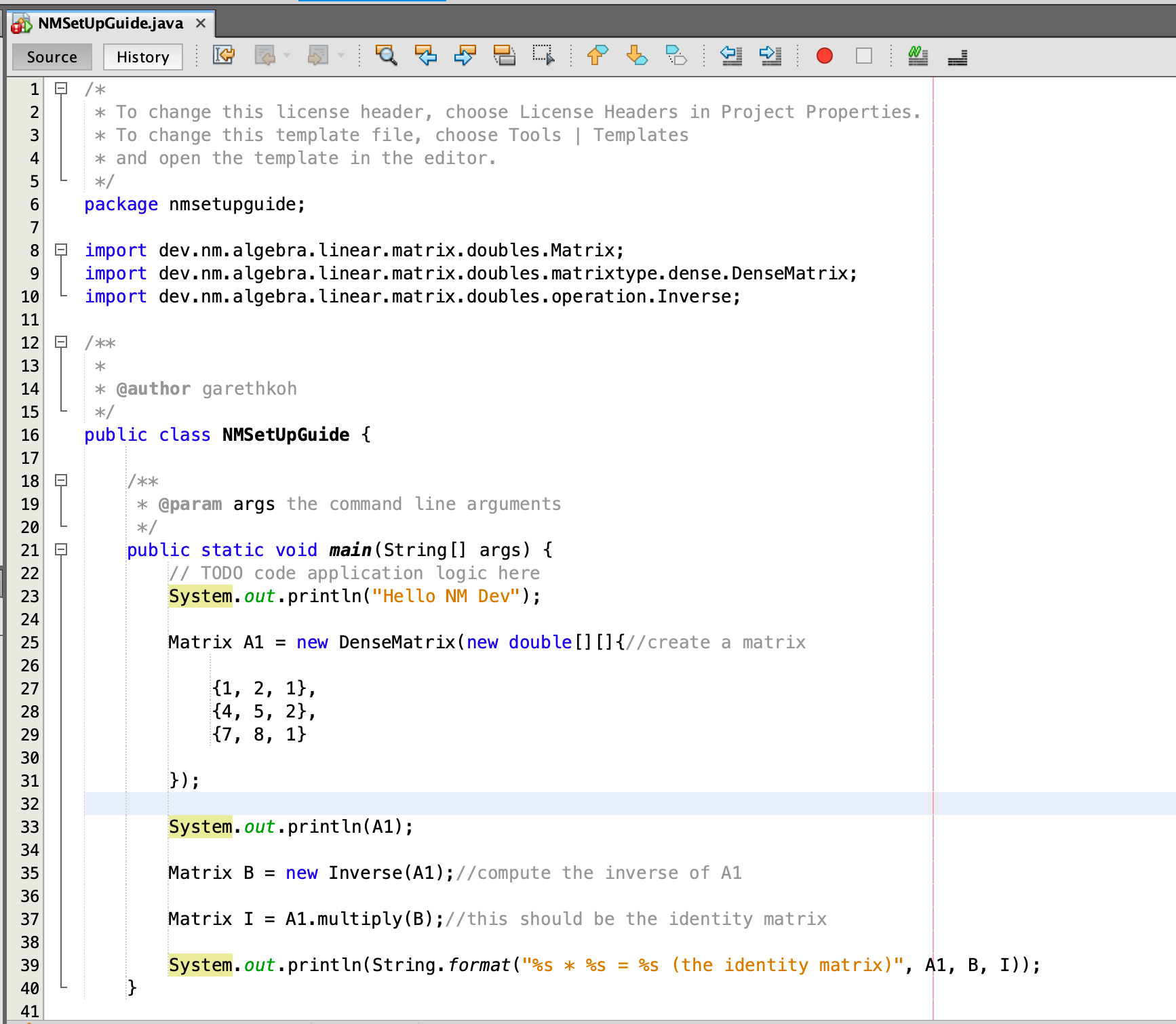[/box]Right click on the project name, e.g., HelloNMDev, and click ‘Run’ (or simply press F6 if you make this project your main project). Voila! You should see this in your output window (Ctrl+4).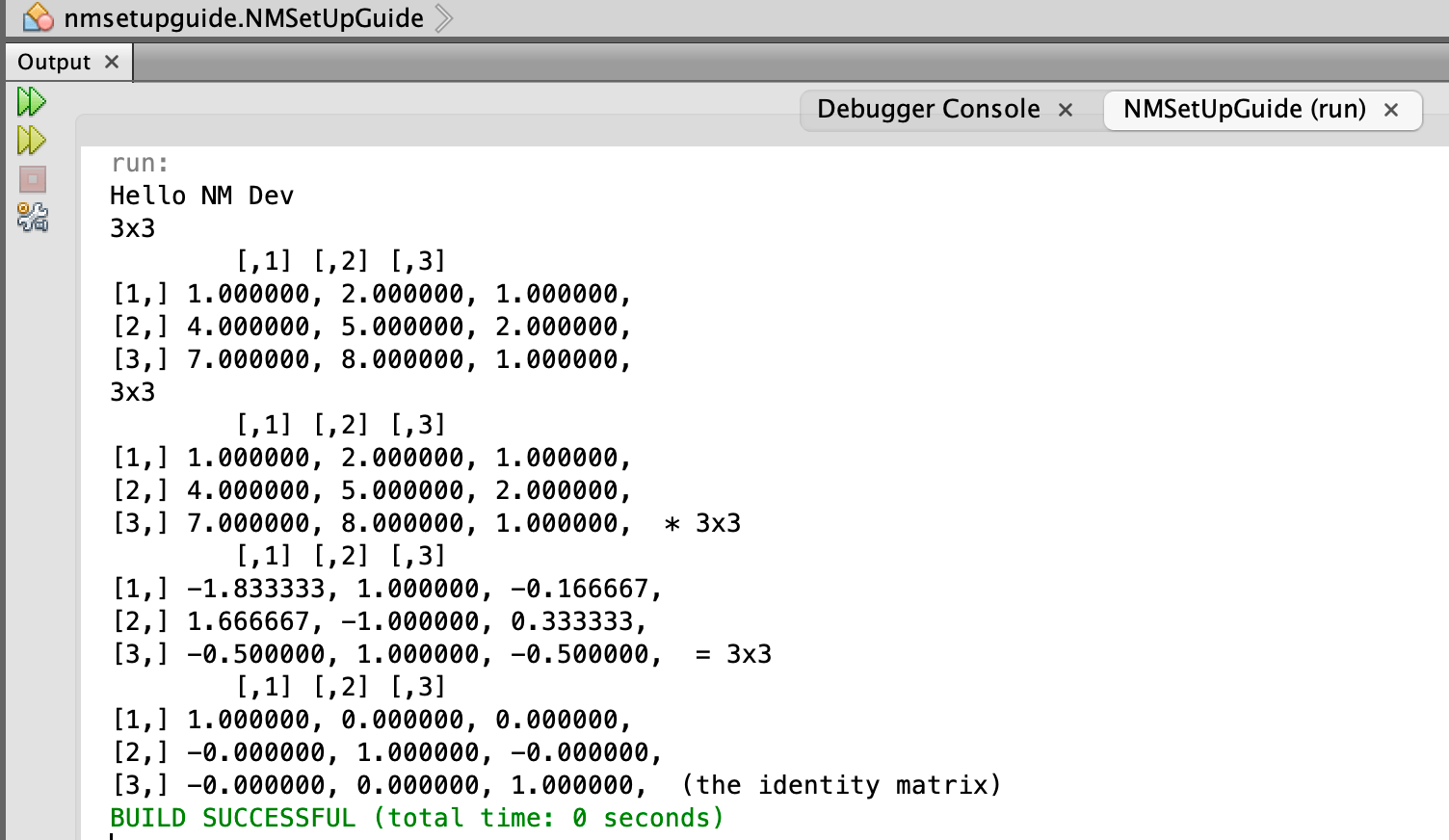Congratulations!

NM Dev’s javadoc is the complete reference to the NM Dev library. You can read it in a browser, e.g., Internet Explorer. You can bring them up dynamically during programming. Each time you press Ctrl+SPACE after the ‘.’ of any object you create, you will see a list of available methods for this object, and the javadoc for the method selected.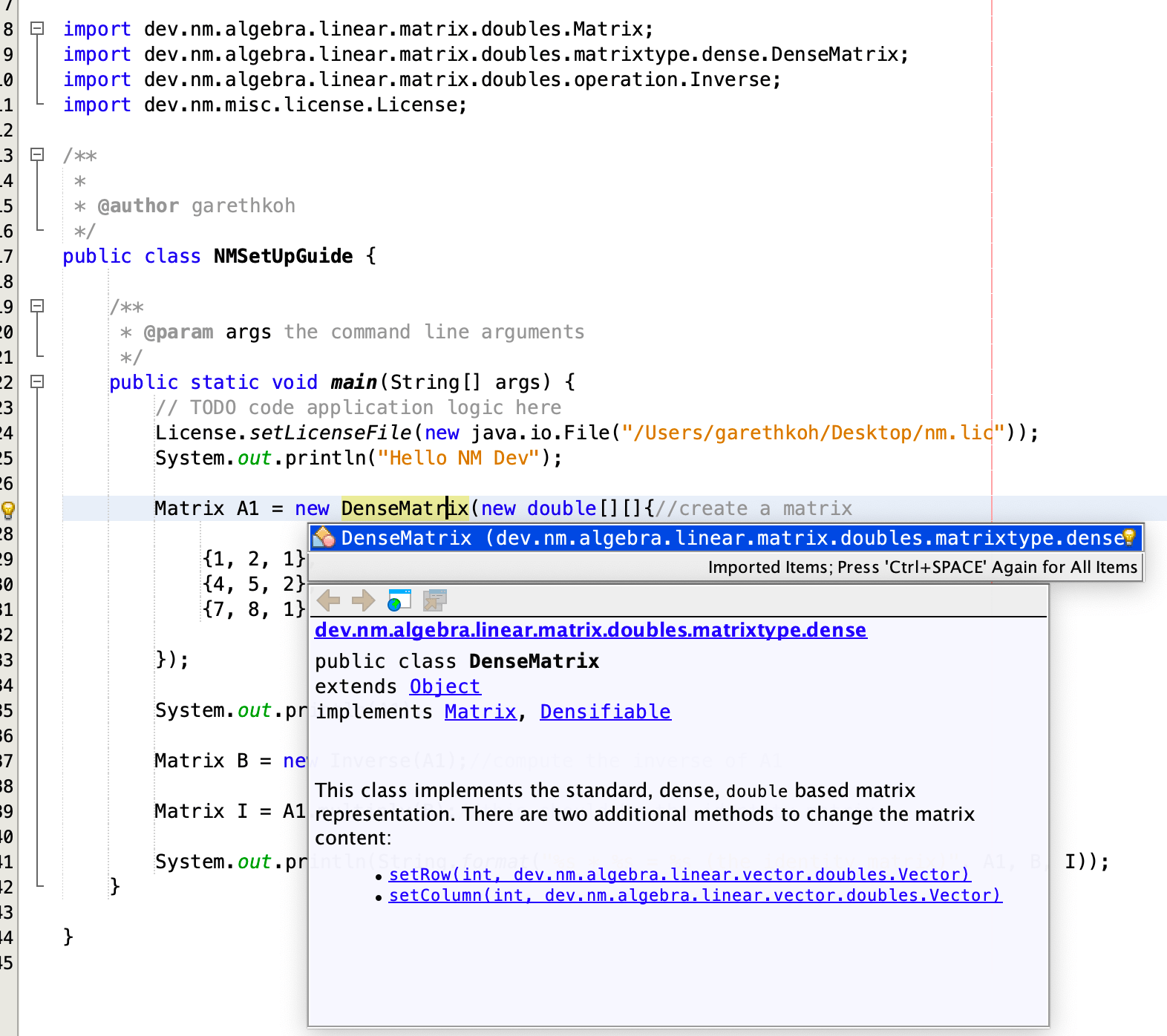## OPDE

This is a collection of numerical algorithms to solve ordinary and partial differential equation problems.

• Ordinary Differential Equation (ODE) solvers for initial value problem (IVP):
• Euler’s method
• Runge Kutta
• 1st order Runge Kutta
• 2nd order Runge Kutta
• 3rd order Runge Kutta
• 4th order Runge Kutta
• 5th order Runge Kutta
• 6th order Runge Kutta
• 7th order Runge Kutta
• 8th order Runge Kutta
• 10th order Runge Kutta
• Runge-Kutta-Fehlberg (RKF45) (adaptive step-size control)
• 1st order
• 2nd order
• 3rd order
• 4th order
• 5th order
• solvers based on Richardson extrapolation
• Burlisch-Stoer extrapolation
• semi-implicit extrapolation (suitable for stiff systems)
• first order system of ODEs
• conversion from high order ODE to first order ODE system
• Partial Differential Equation (PDE) solvers
• finite difference methods:
• elliptic problem:
• iterative central difference method (for Poisson’s equation)
• 1D hyperbolic problem:
• explicit central difference method (for 1D wave equation)
• 2D hyperbolic problem:
• explicit central difference method (for 2D wave equation)
• 1D parabolic problem:
• Crank-Nicolson method (for 1D heat or diffusion equation)
• 2D parabolic problem:
• alternating direction implicit (ADI) method (for 2D heat or diffusion equation)